1. Which of the following would be more appropriate to be replaced with question mark in the following figure?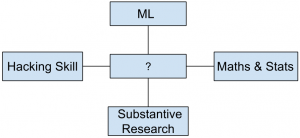a. Data Analysis b. Data Science c. Descriptive Analytics d. None of the mentioned

 2. Which of the following principle is incorrectly represented in the below figure?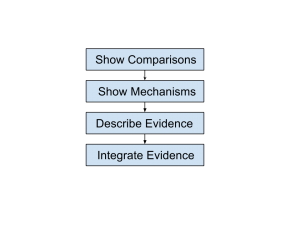a. Show Comparisons b. Integrate Evidence c. Describe Evidence d. None of the mentioned

 3. Data Products shown in the below figure is built using which programming language?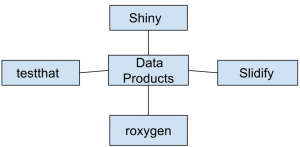a. S b. Python c. R d. Java

 4. Which of the following principle characteristic is odd man out in the below figure?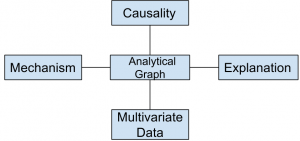a. Principle 1 b. Principle 2 c. Principle 3 d. Principle 4

 5. Which of the following analysis should come in place of the question mark in the below figure?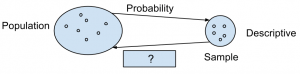a. Inferential b. Exploratory c. Causal d. None of the mentioned

 6. Which of the following type of data science question is missing in the figure?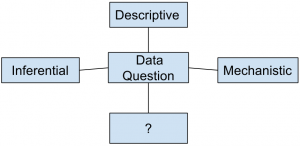a. Correlative b. Exploratory c. Relative d. None of the mentioned

 7. Which of the following term is appropriate to the below figure?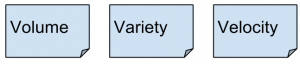a. Large Data b. Big Data c. Dark Data d. None of the mentioned
 8. Which of the following language should be replaced with the question mark in the below figure?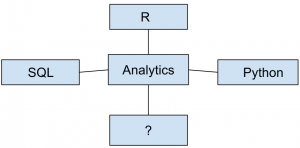a. Java b. PHP c. COBOL d. None of the mentioned
 9. Which of the following design term is perfectly applicable to the below figure?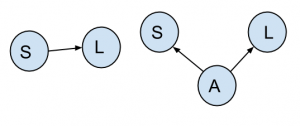a. Correlation b. Confounding c. Causation d. None of the mentioned